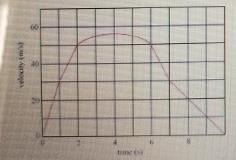# Problem: The graph in the figure (Figure 1) shows the velocity v of a sports car as a function of time t Find the distance d0,2 travelled by the car between t = 0 s and t = 2 s.

###### FREE Expert Solution

Area of a triangle:

$\overline{){\mathbf{A}}{\mathbf{=}}\frac{\mathbf{1}}{\mathbf{2}}{\mathbf{b}}{\mathbf{h}}}$

Area of a trapezium:

a and b are the two parallel sides, while h the separation between them.

87% (167 ratings)###### Problem Details

The graph in the figure (Figure 1) shows the velocity v of a sports car as a function of time tFind the distance d0,2 travelled by the car between t = 0 s and t = 2 s.